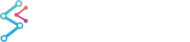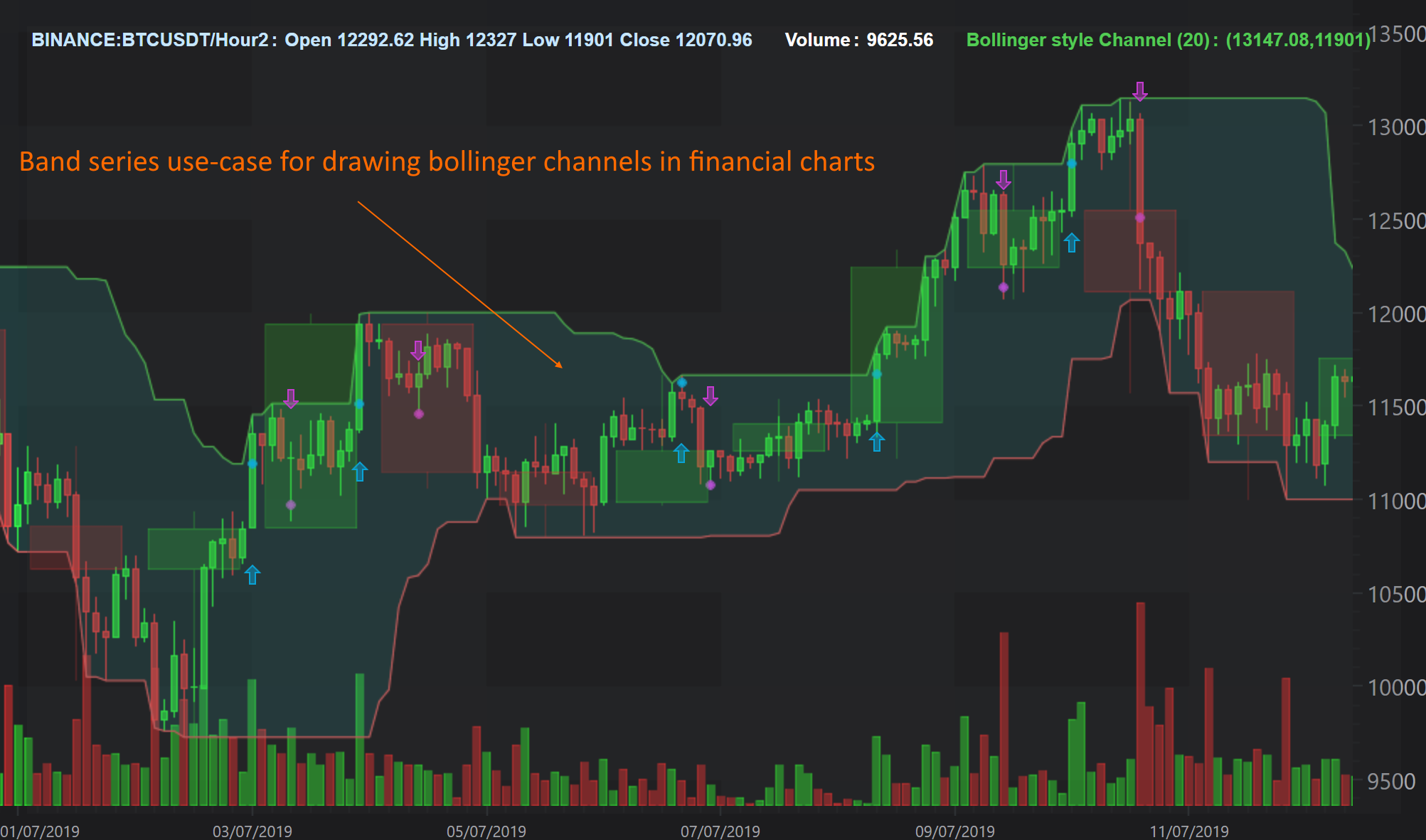﻿ The Band Series type | JavaScript Chart DocumentationSciChart.js JavaScript 2D Charts API > 2D Chart Types > The Band Series type
The Band Series type

A Band Series, or High-Low Fill between two lines can be created using the FastBandRenderableSeries type.

The JavaScript Band Chart Example can be found in the SciChart.Js Examples Suite on Github, or our live demo at demo.scichart.com## Create a Band Series

To create a Javascript Band Chart with SciChart.js, use the following code:

JavaScript Line Chart
Copy Code
```import { XyyDataSeries } from "scichart/Charting/Model/XyyDataSeries";
import { NumericAxis } from "scichart/Charting/Visuals/Axis/NumericAxis";
import { FastBandRenderableSeries } from "scichart/Charting/Visuals/RenderableSeries/FastBandRenderableSeries";
import { SciChartSurface } from "scichart/Charting/Visuals/SciChartSurface";
import { NumberRange } from "scichart/Core/NumberRange";
import { EAxisAlignment } from "scichart/types/AxisAlignment";

// Create a SciChartSurface
const { wasmContext, sciChartSurface } = await SciChartSurface.create(divElementId);
sciChartSurface.xAxes.add(new NumericAxis(wasmContext, { axisAlignment: EAxisAlignment.Top }));
new NumericAxis(wasmContext, { axisAlignment: EAxisAlignment.Left, growBy: new NumberRange(0.4, 0.4) })
);
// The bandseries requires a special dataseries type called XyyDataSeries
// This stores X, Y1, Y2 point data for the two lines in the band
const dataSeries = new XyyDataSeries(wasmContext);
const POINTS = 1000;
const STEP = (3 * Math.PI) / POINTS;
for (let i = 0; i <= 1000; i++) {
const k = 1 - i / 2000;
dataSeries.append(i, Math.sin(i * STEP) * k * 0.7, Math.cos(i * STEP) * k);
}
// Create the band series and add to the chart
const rendSeries = new FastBandRenderableSeries(wasmContext, { dataSeries, strokeThickness: 2 });
rendSeries.fill = "#279B2733";
rendSeries.fillY1 = "#FF191933";
rendSeries.stroke = "#FF1919FF";
rendSeries.strokeY1 = "#279B27FF";
```

In the code above:

## Render a Gap in a Band Series

It is possible to have null points or gaps in a Band Series by passing a data point with a NaN value as the Y value. Please refer to the Common RenderableSeries Features article for more details.

Changing the above code as follows:

JavaScript Discontinuous Line Chart
Copy Code
```const dataSeries = new XyyDataSeries(wasmContext);
const POINTS = 1000;
const STEP = (3 * Math.PI) / POINTS;
for (let i = 0; i <= 1000; i++) {
const k = 1 - i / 2000;
dataSeries.append(i, i % 100 < 20 ? NaN : Math.sin(i * STEP) * k * 0.7, Math.cos(i * STEP) * k);
}
// Create the band series and add to the chart
const rendSeries = new FastBandRenderableSeries(wasmContext, { dataSeries, strokeThickness: 2 });
```

Results in this:## Uses of the Band Series Chart

The Band Series chart primary use is in financial markets, when you want to display things like Bollinger Bands, MACD. For example, our Create Multi Pane Stock Charts demo has a band series for the MACD indicator, which you can see below.Above: a complex visualisation using Band Series. This image is taken from a SciChart WPF sample but all the features are available in SciChart.js as well.

The Band Series Chart can be used to display a mountain or area with a horizontal threshold. For example, if you want to see a series which has a green mountain above zero and red below, to track PnL or profit and loss, you can also use a band series.

For instance, the following code:

Fill above below zero example
Copy Code
```const dataSeries = new XyyDataSeries(wasmContext);
const POINTS = 1000;
const STEP = (3 * Math.PI) / POINTS;
for (let i = 0; i <= 1000; i++) {
const k = 1 - i / 2000;
dataSeries.append(i, 0, Math.sin(i * STEP) * k * 0.7);
}
// Create the band series and add to the chart
const rendSeries = new FastBandRenderableSeries(wasmContext, { dataSeries, strokeThickness: 2 });